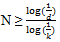Courses

# Test: Butterworth Filters Design - 2

## 10 Questions MCQ Test Digital Signal Processing | Test: Butterworth Filters Design - 2

Description
This mock test of Test: Butterworth Filters Design - 2 for Electrical Engineering (EE) helps you for every Electrical Engineering (EE) entrance exam. This contains 10 Multiple Choice Questions for Electrical Engineering (EE) Test: Butterworth Filters Design - 2 (mcq) to study with solutions a complete question bank. The solved questions answers in this Test: Butterworth Filters Design - 2 quiz give you a good mix of easy questions and tough questions. Electrical Engineering (EE) students definitely take this Test: Butterworth Filters Design - 2 exercise for a better result in the exam. You can find other Test: Butterworth Filters Design - 2 extra questions, long questions & short questions for Electrical Engineering (EE) on EduRev as well by searching above.
QUESTION: 1

### What is the cutoff frequency of the Butterworth filter with a pass band gain KP= -1 dB at ΩP= 4 rad/sec and stop band attenuation greater than or equal to 20dB at ΩS= 8 rad/sec?

Solution:

Explanation: We know that the equation for the cutoff frequency of a Butterworth filter is given as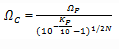We know that KP= -1 dB, ΩP= 4 rad/sec and N=5
Upon substituting the values in the above equation, we get

QUESTION: 2

### What is the system function of the Butterworth filter with specifications as pass band gain KP= -1 dB at ΩP= 4 rad/sec and stop band attenuation greater than or equal to 20dB at ΩS= 8 rad/sec?

Solution:

Explanation: From the given question,
KP= -1 dB, ΩP= 4 rad/sec, KS= -20 dB and ΩS= 8 rad/sec
We find out order as N=5 and Ω_C = 4.5787 rad/sec
We know that for a 5th order normalized low pass Butterworth filter, system equation is given as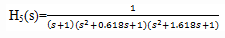The specified low pass filter is obtained by applying low pass-to-low pass transformation on the normalized low pass filter.
That is, Ha(s)=H5(s)|s→s/Ωc
= H5(s)|s→s/4.5787
upon calculating, we get option c.

QUESTION: 3

### If H(s)= 1/(s2+s+1) represent the transfer function of a low pass filter(not Butterworth) with a pass band of 1 rad/sec, then what is the system function of a low pass filter with a pass band 10 rad/sec?

Solution:

Explanation: The low pass-to-low pass transformation is
s→s/Ωu
Hence the required low pass filter is
Ha(s)= H(s)|s→s/10
= 100/(s2+10s+100).

QUESTION: 4

If H(s)= 1/(s2+s+1) represent the transfer function of a low pass filter(not Butterworth) with a pass band of 1 rad/sec, then what is the system function of a high pass filter with a cutoff frequency of 1rad/sec?

Solution:

Explanation: The low pass-to-high pass transformation is
s→Ωu/s
Hence the required high pass filter is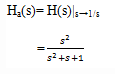QUESTION: 5

If H(s)= 1/(s2+s+1) represent the transfer function of a low pass filter(not Butterworth) with a pass band of 1 rad/sec, then what is the system function of a high pass filter with a cutoff frequency of 10 rad/sec?

Solution:

Explanation: The low pass-to-high pass transformation is
s→Ωu/s
Hence the required low pass filter is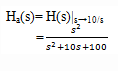QUESTION: 6

If H(s)= 1/(s2+s+1) represent the transfer function of a low pass filter(not Butterworth) with a pass band of 1 rad/sec, then what is the system function of a band pass filter with a pass band of 10 rad/sec and a center frequency of 100 rad/sec?

Solution:

Explanation: The low pass-to-band pass transformation is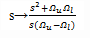Thus the required band pass filter has a transform function as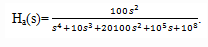QUESTION: 7

If H(s)= 1/(s2+s+1) represent the transfer function of a low pass filter(not Butterworth) with a pass band of 1 rad/sec, then what is the system function of a stop band filter with a stop band of 2 rad/sec and a center frequency of 10 rad/sec?

Solution:

Explanation: The low pass-to- band stop transformation is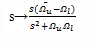Hence the required band stop filter is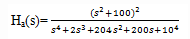QUESTION: 8

What is the stop band frequency of the normalized low pass Butterworth filter used to design a analog band pass filter with -3.0103dB upper and lower cutoff frequency of 50Hz and 20KHz and a stop band attenuation 20dB at 20Hz and 45KHz?

Solution:

Explanation: Given information is
We know that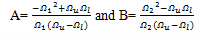=> A= 2.51 and B=2.25

QUESTION: 9

What is the order of the normalized low pass Butterworth filter used to design a analog band pass filter with -3.0103dB upper and lower cutoff frequency of 50Hz and 20KHz and a stop band attenuation 20dB at 20Hz and 45KHz?

Solution:

Explanation: Given information is
We know that
=> A= 2.51 and B=2.25
Hence ΩS= Min{|A|,|B|}=> ΩS=2.25 rad/sec.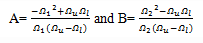The order N of the normalized low pass Butterworth filter is computed as follows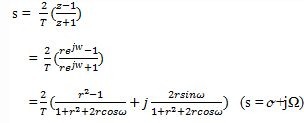= 2.83﻿
Rounding off to the next large integer, we get, N=3.

QUESTION: 10

Which of the following condition is true?

Solution:

Explanation: If ‘d’ is the discrimination factor and ‘K’ is the selectivity factor, then